2019-07-25 17:07:25 qq872890060 阅读数 350
• ###### 人工智能之机器学习算法的介绍

4275 人正在学习 去看看 CSDN讲师

## 八个经典算法

单变量线性回归



## 机器学习

现在的机器学习算法大都分为监督学习和无监督学习，工业界也在用一些半监督学习，就是两者的混合。



## 算法实现思路

### SVM

SVM的构建思路，其实就是将线性回归的判定 边界加粗，寻找一条更为严格的判定边界，这样在训练模型的时候，会获得众多线性可分模型中的最好的那一条，然后在测试集使用的时候，该判定边界适应能力很强，进而使SVM有很强的鲁棒性。

SVM多用于分类问题，也可以用于回归问题。

## 代码实现框架

### 手写底层实现

# 第一部分导入相关的库和包
# 第二部分 读取数据

# 第三部分 数据预处理
def preprocess(data):
# 处理如下，具体要求按题目来做
# 数据提取
# 特征缩放
# 数据初始化
# 数据洗牌
# 数据切割
# 返回数据

# 第四部分 定义激活函数（线性回归不需要）
def g(z):
# 这里我们使用的sigmoid函数
# 神经网络需要返回g()函数的导数

# 第五部分 定义模型
def model():
# 线性回归和逻辑回归使用线性模型
h = X.theta
# 神经网络使用正向传播

# 第六部分定义代价函数
def cost():

# 第七部分 定义梯度下降
# 第一部分
# 数据准备
# 执行梯度下降

# 输出各种数据

# 画图部分 按要求画图



### 调库模块整合

# 导入相关包

# 读取数据

# 数据预处理

# 定义算法模型 （类似于java对象的初始化）

# 模型训练
model.fit()
# 或
model.fit_transform()

# 查看输出各种数据

# 画图部分


## 数据集梳理

数据集：

如果标签值有10的，把10 换成0

对标签进行独热编码



## 评估指标

评估指标：



## 画图部分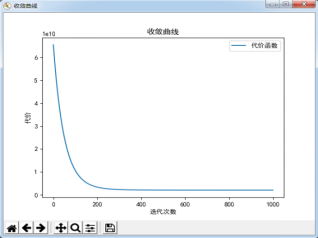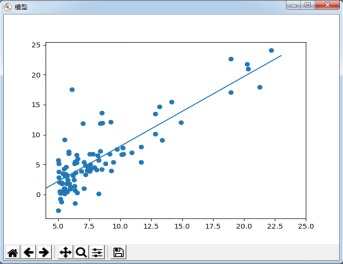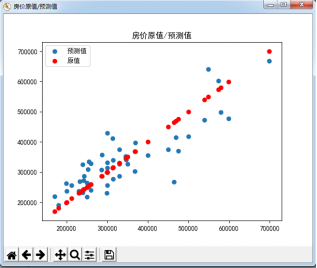## 正则化

正则化：（L1、L2正则）解决过拟合问题

R = λ/2m *np.sum(θ²)   theta0不参与正则化
λ过大，相当于θ小，欠拟合，减小λ
λ过小，相当于θ大，过拟合，增大λ


l2正则化是为原来的代价函数加上所有参数theta的均方和，因为在发生过拟合的时候，曲线过于弯曲会导致某一项的theta值很大，所以加上theta的均方和，会使即使过拟合的代价不大，也会因为正则化项而使代价值变大，大到不符合我们的期望。

2017-04-14 12:08:13 YCM1101743158 阅读数 44928
• ###### 人工智能之机器学习算法的介绍

4275 人正在学习 去看看 CSDN讲师
机器学习无疑是当前数据分析领域的一个热点内容。很多人在平时的工作中都或多或少会用到机器学习的算法。本文为您总结一下常见的机器学习算法，以供您在工作和学习中参考。

机器学习的算法很多。很多时候困惑人们都是，很多算法是一类算法，而有些算法又是从其他算法中延伸出来的。这里，我们从两个方面来给大家介绍，第一个方面是学习的方式，第二个方面是算法的类似性。

## 一、  决策树优点

1、决策树易于理解和解释，可以可视化分析，容易提取出规则。

2、可以同时处理标称型和数值型数据。

3、测试数据集时，运行速度比较快。

4、决策树可以很好的扩展到大型数据库中，同时它的大小独立于数据库大小。

## 二、决策树缺点

1、对缺失数据处理比较困难。

2、容易出现过拟合问题。

3、忽略数据集中属性的相互关联。

4、ID3算法计算信息增益时结果偏向数值比较多的特征。

## 三、改进措施

1、对决策树进行剪枝。可以采用交叉验证法和加入正则化的方法。

2、使用基于决策树的combination算法，如bagging算法，randomforest算法，可以解决过拟合的问题

# KNN算法

## 一、KNN算法的优点

1、KNN是一种在线技术，新数据可以直接加入数据集而不必进行重新训练

2、KNN理论简单，容易实现

## 二、KNN算法的缺点

1、对于样本容量大的数据集计算量比较大。

2、样本不平衡时，预测偏差比较大。如：某一类的样本比较少，而其它类样本比较多。

3、KNN每一次分类都会重新进行一次全局运算。

4、k值大小的选择。

# 支持向量机（SVM）

## 一、  SVM优点

1、解决小样本下机器学习问题。

2、解决非线性问题。

3、无局部极小值问题。（相对于神经网络等算法）

4、可以很好的处理高维数据集。

5、泛化能力比较强。

## 二、SVM缺点

1、对于核函数的高维映射解释力不强，尤其是径向基函数。

2、对缺失数据敏感。

## 三、SVM应用领域

1、很好的利用了弱分类器进行级联。

2、可以将不同的分类算法作为弱分类器。

2、数据不平衡导致分类精度下降。

3、训练比较耗时，每次重新选择当前分类器最好切分点。

# 朴素贝叶斯算法

## 一、  朴素贝叶斯算法优点

1、对大数量训练和查询时具有较高的速度。即使使用超大规模的训练集，针对每个项目通常也只会有相对较少的特征数，并且对项目的训练和分类也仅仅是特征概率的数学运算而已。

2、支持增量式运算。即可以实时的对新增的样本进行训练。

3、朴素贝叶斯对结果解释容易理解。

## 二、朴素贝叶斯缺点

1、由于使用了样本属性独立性的假设，所以如果样本属性有关联时其效果不好。

# Logistic回归算法

1、计算代价不高，易于理解和实现

1、容易产生欠拟合。

2、分类精度不高。

## 三、logistic回归应用领域

Logistic回归的扩展softmax可以应用于多分类领域，如手写字识别等。

# 人工神经网络

## 一、  神经网络优点

1、分类准确度高，学习能力极强。

2、对噪声数据鲁棒性和容错性较强。

3、有联想能力，能逼近任意非线性关系。

## 二、神经网络缺点

1、神经网络参数较多，权值和阈值。

2、黑盒过程，不能观察中间结果。

3、学习过程比较长，有可能陷入局部极小值。

## 三、人工神经网络应用领域

===============================================================================================

1. 首先是SVM。因为我做的文本处理比较多，所以比较熟悉SVM。SVM也叫支持向量机，其把数据映射到多维空间中以点的形式存在，然后找到能够分类的最优超平面，最后根据这个平面来分类。SVM能对训练集之外的数据做很好的预测、泛化错误率低、计算开销小、结果易解释，但其对参数调节和核函数的参数过于敏感。个人感觉SVM是二分类的最好的方法，但也仅限于二分类。如果要使用SVM进行多分类，也是在向量空间中实现多次二分类。
SVM有一个核心函数SMO，也就是序列最小最优化算法。SMO基本是最快的二次规划优化算法，其核心就是找到最优参数α，计算超平面后进行分类。SMO方法可以将大优化问题分解为多个小优化问题求解，大大简化求解过程。某些条件下，把原始的约束问题通过拉格朗日函数转化为无约束问题，如果原始问题求解棘手，在满足KKT的条件下用求解对偶问题来代替求解原始问题，使得问题求解更加容易。 SVM还有一个重要函数是核函数。核函数的主要作用是将数据从低位空间映射到高维空间。详细的内容我就不说了，因为内容实在太多了。总之，核函数可以很好的解决数据的非线性问题，而无需考虑映射过程。

2. 第二个是KNN。KNN将测试集的数据特征与训练集的数据进行特征比较，然后算法提取样本集中特征最近邻数据的分类标签，即KNN算法采用测量不同特征值之间的距离的方法进行分类。KNN的思路很简单，就是计算测试数据与类别中心的距离。KNN具有精度高、对异常值不敏感、无数据输入假定、简单有效的特点，但其缺点也很明显，计算复杂度太高。要分类一个数据，却要计算所有数据，这在大数据的环境下是很可怕的事情。而且，当类别存在范围重叠时，KNN分类的精度也不太高。所以，KNN比较适合小量数据且精度要求不高的数据。
KNN有两个影响分类结果较大的函数，一个是数据归一化，一个是距离计算。如果数据不进行归一化，当多个特征的值域差别很大的时候，最终结果就会受到较大影响；第二个是距离计算。这应该算是KNN的核心了。目前用的最多的距离计算公式是欧几里得距离，也就是我们常用的向量距离计算方法。
个人感觉，KNN最大的作用是可以随时间序列计算，即样本不能一次性获取只能随着时间一个一个得到的时候，KNN能发挥它的价值。至于其他的特点，它能做的，很多方法都能做；其他能做的它却做不了。

3. 第三个就是Naive Bayes了。Naive Bayes简称NB（牛X），为啥它牛X呢，因为它是基于Bayes概率的一种分类方法。贝叶斯方法可以追溯到几百年前，具有深厚的概率学基础，可信度非常高。Naive Baye中文名叫朴素贝叶斯，为啥叫“朴素”呢？因为其基于一个给定假设：给定目标值时属性之间相互条件独立。比如我说“我喜欢你”，该假设就会假定“我”、“喜欢”、“你”三者之间毫无关联。仔细想想，这几乎是不可能的。马克思告诉我们：事物之间是有联系的。同一个事物的属性之间就更有联系了。所以，单纯的使用NB算法效率并不高，大都是对该方法进行了一定的改进，以便适应数据的需求。
NB算法在文本分类中用的非常多，因为文本类别主要取决于关键词，基于词频的文本分类正中NB的下怀。但由于前面提到的假设，该方法对中文的分类效果不好，因为中文顾左右而言他的情况太多，但对直来直去的老美的语言，效果良好。至于核心算法嘛，主要思想全在贝叶斯里面了，没啥可说的。

4. 第四个是回归。回归有很多，Logistic回归啊、岭回归啊什么的，根据不同的需求可以分出很多种。这里我主要说说Logistic回归。为啥呢？因为Logistic回归主要是用来分类的，而非预测。回归就是将一些数据点用一条直线对这些点进行拟合。而Logistic回归是指根据现有数据对分类边界线建立回归公式，以此进行分类。该方法计算代价不高，易于理解和实现，而且大部分时间用于训练，训练完成后分类很快；但它容易欠拟合，分类精度也不高。主要原因就是Logistic主要是线性拟合，但现实中很多事物都不满足线性的。即便有二次拟合、三次拟合等曲线拟合，也只能满足小部分数据，而无法适应绝大多数数据，所以回归方法本身就具有局限性。但为什么还要在这里提出来呢？因为回归方法虽然大多数都不合适，但一旦合适，效果就非常好。
Logistic回归其实是基于一种曲线的，“线”这种连续的表示方法有一个很大的问题，就是在表示跳变数据时会产生“阶跃”的现象，说白了就是很难表示数据的突然转折。所以用Logistic回归必须使用一个称为“海维塞德阶跃函数”的Sigmoid函数来表示跳变。通过Sigmoid就可以得到分类的结果。
为了优化Logistic回归参数，需要使用一种“梯度上升法”的优化方法。该方法的核心是，只要沿着函数的梯度方向搜寻，就可以找到函数的最佳参数。但该方法在每次更新回归系数时都需要遍历整个数据集，对于大数据效果还不理想。所以还需要一个“随机梯度上升算法”对其进行改进。该方法一次仅用一个样本点来更新回归系数，所以效率要高得多。

5. 第五个是决策树。据我了解，决策树是最简单，也是曾经最常用的分类方法了。决策树基于树理论实现数据分类，个人感觉就是数据结构中的B+树。决策树是一个预测模型，他代表的是对象属性与对象值之间的一种映射关系。决策树计算复杂度不高、输出结果易于理解、对中间值缺失不敏感、可以处理不相关特征数据。其比KNN好的是可以了解数据的内在含义。但其缺点是容易产生过度匹配的问题，且构建很耗时。决策树还有一个问题就是，如果不绘制树结构，分类细节很难明白。所以，生成决策树，然后再绘制决策树，最后再分类，才能更好的了解数据的分类过程。
决策树的核心树的分裂。到底该选择什么来决定树的分叉是决策树构建的基础。最好的方法是利用信息熵实现。熵这个概念很头疼，很容易让人迷糊，简单来说就是信息的复杂程度。信息越多，熵越高。所以决策树的核心是通过计算信息熵划分数据集。

===================================================

http://blog.csdn.NET/vola9527/article/details/43347747

1）C4.5算法：

ID3算法是以信息论为基础，以信息熵和信息增益度为衡量标准，从而实现对数据的归纳分类。ID3算法计算每个属性的信息增益，并选取具有最高增益的属性作为给定的测试属性。

C4.5算法核心思想是ID3算法，是ID3算法的改进，改进方面有：

1）用信息增益率来选择属性，克服了用信息增益选择属性时偏向选择取值多的属性的不足；

2）在树构造过程中进行剪枝

3）能处理非离散的数据

4）能处理不完整的数据

C4.5算法优点：产生的分类规则易于理解，准确率较高。

1)在构造树的过程中，需要对数据集进行多次的顺序扫描和排序，因而导致算法的低效。

2)C4.5只适合于能够驻留于内存的数据集，当训练集大得无法在内存容纳时程序无法运行。

2）K means 算法

3）朴素贝叶斯算法：

4)K最近邻分类算法（KNN）

1）K值需要预先设定，而不能自适应

2）当样本不平衡时，如一个类的样本容量很大，而其他类样本容量很小时，有可能导致当输入一个新样本时，该样本的K个邻居中大容量类的样本占多数。

5)EM最大期望算法

EM算法是基于模型的聚类方法，是在概率模型中寻找参数最大似然估计的算法，其中概率模型依赖于无法观测的隐藏变量。E步估计隐含变量，M步估计其他参数，交替将极值推向最大。

EM算法比K-means算法计算复杂，收敛也较慢，不适于大规模数据集和高维数据，但比K-means算法计算结果稳定、准确。EM经常用在机器学习和计算机视觉的数据集聚（Data Clustering）领域。

6）PageRank算法

1）PageRank算法忽略了网页搜索的时效性。

1. 先通过对N个训练样本的学习得到第一个弱分类器；
2. 将分错的样本和其他的新数据一起构成一个新的N个的训练样本，通过对这个样本的学习得到第二个弱分类器；
3. 将和都分错了的样本加上其他的新样本构成另一个新的N个的训练样本，通过对这个样本的学习得到第三个弱分类器；
4. 如此反复，最终得到经过提升的强分类器。

8）Apriori算法

Apriori算法是一种挖掘关联规则的算法，用于挖掘其内含的、未知的却又实际存在的数据关系，其核心是基于两阶段频集思想的递推算法 。

Apriori算法分为两个阶段：

1）寻找频繁项集

2）由频繁项集找关联规则

1） 在每一步产生侯选项目集时循环产生的组合过多，没有排除不应该参与组合的元素；

2） 每次计算项集的支持度时，都对数据库中    的全部记录进行了一遍扫描比较，需要很大的I/O负载。

9）SVM支持向量机

SVM在解决小样本、非线性及高维模式识别问题中表现出许多特有的优势，并能够推广应用到函数拟合等其他机器学习问题中。

10）CART分类与回归树

1）非常灵活，可以允许有部分错分成本，还可指定先验概率分布，可使用自动的成本复杂性剪枝来得到归纳性更强的树。

2）在面对诸如存在缺失值、变量数多等问题时CART 显得非常稳健。

2016-08-21 23:26:48 JIEJINQUANIL 阅读数 32349
• ###### 人工智能之机器学习算法的介绍

4275 人正在学习 去看看 CSDN讲师

机器学习无疑是当前数据分析领域的一个热点内容。很多人在平时的工作中都或多或少会用到机器学习的算法。本文总结一下常见的机器学习算法，以供参考。机器学习的算法很多，很多算法是一类算法，而有些算法又是从其他算法中延伸出来的。这里从两个方面进行总结，第一个方面是学习的方式，第二个方面是算法的类似性。

根据数据类型的不同，对一个问题的建模有不同的方式。在机器学习或者人工智能领域，人们首先会考虑算法的学习方式。将算法按照学习方式分类是一个不错的想法，这样可以让人们在建模和算法选择的时候考虑能根据输入数据来选择最合适的算法来获得最好的结果。在机器学习领域，有几种主要的学习方式：监督学习非监督学习半监督学习强化学习

### 1、监督式学习：在监督式学习下，输入数据被称为“训练数据”，每组训练数据有一个明确的标识或结果，如对防垃圾邮件系统中“垃圾邮件”“非垃圾邮件”，对手写数字识别中的“1“，”2“，”3“，”4“等。在建立预测模型的时候，监督式学习建立一个学习过程，将预测结果与“训练数据”的实际结果进行比较，不断的调整预测模型，直到模型的预测结果达到一个预期的准确率。监督式学习的常见应用场景如分类问题和回归问题。常见监督式学习算法有决策树学习(ID3,C4.5等)，朴素贝叶斯分类，最小二乘回归，逻辑回归（Logistic Regression），支撑矢量机，集成方法以及反向传递神经网络（Back Propagation Neural Network）等等。

### 2、非监督式学习：在非监督式学习中，数据并不被特别标识，学习模型是为了推断出数据的一些内在结构。常见的应用场景包括关联规则的学习以及聚类等。常见非监督学习算法包括奇异值分解、主成分分析，独立成分分析，Apriori算法以及k-Means算法等等。

### 3、半监督式学习：在此学习方式下，输入数据部分被标识，部分没有被标识，这种学习模型可以用来进行预测，但是模型首先需要学习数据的内在结构以便合理的组织数据来进行预测。应用场景包括分类和回归，算法包括一些对常用监督式学习算法的延伸，这些算法首先试图对未标识数据进行建模，在此基础上再对标识的数据进行预测。如图论推理算法（Graph Inference）或者拉普拉斯支持向量机（Laplacian SVM）等。

### 4、强化学习：在这种学习模式下，输入数据作为对模型的反馈，不像监督模型那样，输入数据仅仅是作为一个检查模型对错的方式，在强化学习下，输入数据直接反馈到模型，模型必须对此立刻作出调整。常见的应用场景包括动态系统以及机器人控制等。常见算法包括Q-Learning以及时间差学习（Temporal difference learning）。在企业数据应用的场景下， 人们最常用的可能就是监督式学习和非监督式学习的模型。 在图像识别等领域，由于存在大量的非标识的数据和少量的可标识数据， 目前半监督式学习是一个很热的话题。 而强化学习更多的应用在机器人控制及其他需要进行系统控制的领域。

## 二、算法类似性

根据算法的功能和形式的类似性，我们可以把算法分类，比如说基于树的算法，基于神经网络的算法等等。当然，机器学习的范围非常庞大，有些算法很难明确归类到某一类。而对于有些分类来说，同一分类的算法可以针对不同类型的问题。这里，我们尽量把常用的算法按照最容易理解的方式进行分类。

### 1、回归算法：回归算法是试图采用对误差的衡量来探索变量之间的关系的一类算法。回归算法是统计机器学习的利器。在机器学习领域，人们说起回归，有时候是指一类问题，有时候是指一类算法，这一点常常会使初学者有所困惑。常见的回归算法包括：最小二乘法（Ordinary Least Square），逻辑回归（Logistic Regression），逐步式回归（Stepwise Regression），多元自适应回归样条（Multivariate Adaptive Regression Splines）以及本地散点平滑估计（Locally Estimated Scatterplot Smoothing）。

通常，回归可以被用于在现实世界的应用，如：

• 信用评分
• 度量营销活动的成功率
• 预测某一产品的收入
• 在一个特定的日子里会发生地震吗？

### 2、基于实例的算法基于实例的算法常常用来对决策问题建立模型，这样的模型常常先选取一批样本数据，然后根据某些近似性把新数据与样本数据进行比较。通过这种方式来寻找最佳的匹配。因此，基于实例的算法常常也被称为“赢家通吃”学习或者“基于记忆的学习”。常见的算法包括 k-Nearest Neighbor(KNN), 学习矢量量化（Learning Vector Quantization， LVQ），以及自组织映射算法（Self-Organizing Map ， SOM）。

### 3、正则化方法正则化方法是其他算法（通常是回归算法）的延伸，根据算法的复杂度对算法进行调整。正则化方法通常对简单模型予以奖励而对复杂算法予以惩罚。常见的算法包括：Ridge Regression， Least Absolute Shrinkage and Selection Operator（LASSO），以及弹性网络（Elastic Net）。

### 4、决策树学习决策树算法根据数据的属性采用树状结构建立决策模型， 决策树模型常常用来解决分类和回归问题。常见的算法包括：分类及回归树（Classification And Regression Tree， CART）， ID3 (Iterative Dichotomiser 3)， C4.5， Chi-squared Automatic Interaction Detection(CHAID), Decision Stump, 随机森林（Random Forest）， 多元自适应回归样条（MARS）以及梯度推进机（Gradient Boosting Machine， GBM）。

### 5、贝叶斯方法贝叶斯方法算法是基于贝叶斯定理的一类算法，主要用来解决分类和回归问题。常见算法包括：朴素贝叶斯算法，平均单依赖估计（Averaged One-Dependence Estimators， AODE），以及Bayesian Belief Network（BBN）。

一些现实中的例子：

• 标记一个电子邮件为垃圾邮件或非垃圾邮件
• 将新闻文章分为技术类、政治类或体育类
• 检查一段文字表达积极的情绪，或消极的情绪？
• 用于人脸识别软件

### 6、基于核的算法基于核的算法中最著名的莫过于支持向量机（SVM）了。 基于核的算法把输入数据映射到一个高阶的向量空间， 在这些高阶向量空间里， 有些分类或者回归问题能够更容易的解决。 常见的基于核的算法包括：支持向量机（Support Vector Machine， SVM）， 径向基函数（Radial Basis Function ，RBF)， 以及线性判别分析（Linear Discriminate Analysis ，LDA)等。

就规模而言，其中一些最主要的问题已经使用支持向量机解决了（通过适当的修改），如，入广告显示，人类的剪接位点识别，基于图像的性别检测，大规模图像分类等等。

### 7、聚类算法聚类，就像回归一样，有时候人们描述的是一类问题，有时候描述的是一类算法。聚类算法通常按照中心点或者分层的方式对输入数据进行归并。所以的聚类算法都试图找到数据的内在结构，以便按照最大的共同点将数据进行归类。常见的聚类算法包括 k-Means算法以及期望最大化算法（Expectation Maximization， EM）。• 基于质心的算法
• 基于连通性的算法
• 基于密度的算法
• 概率聚类
• 降维
• 神经网络/深度学习

### 8、关联规则学习关联规则学习通过寻找最能够解释数据变量之间关系的规则，来找出大量多元数据集中有用的关联规则。常见算法包括 Apriori算法和Eclat算法等。

### 9、人工神经网络人工神经网络算法模拟生物神经网络，是一类模式匹配算法。通常用于解决分类和回归问题。人工神经网络是机器学习的一个庞大的分支，有几百种不同的算法。（其中深度学习就是其中的一类算法，我们会单独讨论），重要的人工神经网络算法包括：感知器神经网络（Perceptron Neural Network）, 反向传递（Back Propagation）， Hopfield网络，自组织映射（Self-Organizing Map, SOM）。学习矢量量化（Learning Vector Quantization， LVQ）。

### 10、深度学习深度学习算法是对人工神经网络的发展。 在近期赢得了很多关注， 特别是百度也开始发力深度学习后， 更是在国内引起了很多关注。   在计算能力变得日益廉价的今天，深度学习试图建立大得多也复杂得多的神经网络。很多深度学习的算法是半监督式学习算法，用来处理存在少量未标识数据的大数据集。常见的深度学习算法包括：受限波尔兹曼机（Restricted Boltzmann Machine， RBN）， Deep Belief Networks（DBN），卷积网络（Convolutional Network）, 堆栈式自动编码器（Stacked Auto-encoders）。

### 11、降低维度算法像聚类算法一样，降低维度算法试图分析数据的内在结构，不过降低维度算法是以非监督学习的方式试图利用较少的信息来归纳或者解释数据。这类算法可以用于高维数据的可视化或者用来简化数据以便监督式学习使用。常见的算法包括：主成份分析（Principle Component Analysis， PCA），偏最小二乘回归（Partial Least Square Regression，PLS）， Sammon映射，多维尺度（Multi-Dimensional Scaling, MDS）,  投影追踪（Projection Pursuit）等。PCA降维示意图其中，ICA 和 PCA 是相关的，但是它是一种更强大的技术，当那些经典的方法完全失效的时候，它能够从数据源中发现潜在的因素。它的应用包括数字图像，文档数据库，经济指标和心理测量。

### 12、集成算法：• 他们拉平了输出偏差：如果你将具有民主党倾向的民意调查和具有共和党倾向的民意调查取平均，你将得到一个中和的没有倾向一方的结果。
• 它们减小了方差：一堆模型的聚合结果和单一模型的结果相比具有更少的噪声。在金融领域，这被称为多元化——多只股票的混合投资要比一只股票变化更小。这就是为什么数据点越多你的模型会越好，而不是数据点越少越好。
• 它们不太可能产生过拟合：如果你有一个单独的没有过拟合的模型，你是用一种简单的方式（平均，加权平均，逻辑回归）将这些预测结果结合起来，然后就没有产生过拟合的空间了。

2018-08-09 15:24:29 thormas1996 阅读数 510
• ###### 人工智能之机器学习算法的介绍

4275 人正在学习 去看看 CSDN讲师

# 机器学习算法总结

## 1. 朴素贝叶斯模型

$P\left(y|x\right)=\frac{P\left(x|y\right)P\left(y\right)}{P\left(x\right)}$${P(y|x) = \frac{P(x|y) P(y)} {P(x)} }$

P(y|x)是后验概率，P(x|y) 是似然概率，P(y)是先验概率

$P\left(类别|特征\right)=\frac{P\left(特征|类别\right)P\left(类别\right)}{P\left(特征\right)}$${P(类别|特征) = \frac{P(特征|类别)P(类别)}{P(特征)}}$

$y=argma{x}_{y}P\left(y|x\right)=argma{x}_{y}P\left(x|y\right)P\left(y\right)$${y = argmax_y P(y|x) = argmax_y P(x|y) P(y) }$

• 优点
对小规模的数据表现很好，能处理多分类任务。
对缺失数据不太敏感，算法也比较简单，常用于文本分类。
当样本很多时，可以分批训练，即可以增量式训练。

• 缺点
对于属性较多或者属性之间关联性较大的情况，效果很差，容易欠拟合。
需要计算先验概率，先验模型对预测结果有很大影响。
由于通过先验和条件概率计算后验概率，所以分类决策存在一定的错误率。

• 改进
先对关联性大的属性聚类，再用朴素贝叶斯分类；

## 2. 逻辑回归

$y=\frac{1}{1+{e}^{-z}}$${y = \frac{1}{1+e^{-z}}}$

$ln\frac{y}{1-y}=z={w}^{T}x+b$${ln\frac{y}{1-y}=z=w^Tx+b}$

$P\left(y=1|x\right)=\frac{1}{1+{e}^{-z}}=\varphi \left(z\right)$${P(y=1|x) = \frac{1}{1+e^{-z}} = \phi(z)}$

$P\left(y=0|x\right)=\frac{{e}^{-z}}{1+{e}^{-z}}=1-\varphi \left(z\right)$${P(y=0|x) = \frac{e^{-z}}{1+e^{-z}} = 1-\phi(z)}$

${l}_{y}=\varphi \left(z{\right)}^{y}×\left(1-\varphi \left(z\right){\right)}^{1-y}$${ l_y = \phi(z)^y \times (1-\phi(z))^{1-y}}$

$L=-\sum _{y}ln{l}_{y}=\sum _{y}-yln\varphi \left(z\right)-\left(1-y\right)ln\left(1-\varphi \left(z\right)\right)$${L = -\sum_y ln l_y = \sum_y -y ln\phi(z)-(1-y)ln(1-\phi(z))}$

- 优点

• 缺点
当特征空间很大时，逻辑回归的性能不是很好。
因为模型简单，所以容易欠拟合，一般准确度不太高。
不能很好地处理大量多类特征或变量。
只能处理两分类问题（在此基础上衍生出来的softmax可以用于多分类），且必须线性可分。

## 3. KNN（K近邻）

• 优点
精度高
对异常值不敏感
无数据输入假定
简单有效，并且可以随时加入新样本

• 缺点
复杂度太高，计算成本大，需要大量内存
当类别存在范围重叠时，KNN分类的精度不太高

## 4. 感知机

$f\left(x\right)=sign\left(wx+b\right)$${f(x) = sign(wx+b)}$

$w\left(t\right)=w\left(t-1\right)+{y}_{i}{x}_{i}$${w(t) = w(t-1) + y_ix_i}$

$b\left(t\right)=b\left(t-1\right)+{y}_{i}$${b(t) = b(t-1) + y_i}$

## 5. SVM

${\omega }^{T}x+b=0$${\omega^Tx+b=0}$

${\omega }^{T}x+b=1$${\omega^Tx+b=1}$

${\omega }^{T}x+b=-1$${\omega^Tx+b=-1}$

$max\frac{2}{||\omega ||}$${max \frac{2}{||\omega||}}$

$min\frac{1}{2}||\omega |{|}^{2}$${min \frac{1}{2} ||\omega||^2}$

$s.t.{y}_{i}\left({\omega }^{T}{x}_{i}+b\right)\ge 1$${s.t. y_i(\omega^Tx_i+b) \geq 1}$

$L=\frac{1}{2}||\omega |{|}^{2}+\sum _{i=1}^{m}{\alpha }_{i}\left(1-{y}_{i}\left({\omega }^{T}{x}_{i}+b\right)\right)$${L = \frac{1}{2}||\omega||^2 +\sum\limits_{i=1}^m \alpha_i (1-y_i(\omega^Tx_i+b))}$

$\omega$${\omega}$和b求偏导为零，得到：

$\omega =\sum _{i=1}^{m}{\alpha }_{i}{y}_{i}{x}_{i}$${\omega =\sum\limits_{i=1}^m \alpha_i y_i x_i }$

$\sum _{i=1}^{m}{\alpha }_{i}{y}_{i}=0$${\sum\limits_{i=1}^m \alpha_i y_i = 0}$

$max\sum _{i=1}^{m}{\alpha }_{i}-\frac{1}{2}\sum _{i=1}^{m}\sum _{j=1}^{m}{\alpha }_{i}{\alpha }_{j}{y}_{i}{y}_{j}{x}_{i}^{T}{x}_{j}$${max \sum\limits_{i=1}^m \alpha_i - \frac{1}{2} \sum\limits_{i=1}^m \sum\limits_{j=1}^m \alpha_i \alpha_j y_i y_j x_i^T x_j}$

$s.t.\sum _{i=1}^{m}{\alpha }_{i}{y}_{i}=0$${s.t. \sum\limits_{i=1}^m \alpha_i y_i = 0}$

$y\left(x\right)={\omega }^{T}x+b$${y(x) = \omega^T x + b}$

KKT 条件：
${\alpha }_{i}\ge 0$${\alpha_i \geq 0}$
${y}_{i}f\left({x}_{1}\right)-1\ge 0$${y_if(x_1)-1 \geq 0}$
${\alpha }_{i}\left({y}_{i}f\left({x}_{i}\right)-1\right)=0$${\alpha_i (y_if(x_i)-1) = 0}$

• 优点
可以解决高维问题，即大型特征空间。
能够处理非线性特征的相互作用。
无需依赖整个数据。
可以提高泛化能力。

• 缺点
当观测样本很多时，效率并不是很高。
对非线性问题没有通用解决方案，有时候很难找到一个合适的核函数。
对缺失数据敏感。

## 5. 决策树

$Entropy=-\sum {p}_{k}lo{g}_{2}{p}_{k}$${Entropy = - \sum p_k log_2 p_k}$
$Gini=1-\sum {p}_{k}^{2}$${Gini = 1 - \sum p_k^2}$

- 优点

• 缺点
容易发生过拟合（随机森林可以很大程度上减少过拟合）。
忽略了数据之间的相关性。
对于那些各类别样本数量不一致的数据，在决策树当中,信息增益的结果偏向于那些具有更多数值的特征（只要是使用了信息增益，都有这个缺点，如RF）。

## 6. 集成学习

boosting & bagging
boosting 是每次迭代会给错误的样本更高的权重使得下一个弱分类器能正确观察到错的地方。最后由几个弱分类器加起来，根据准确率给每个分类器一个投票的权重。
bagging 是每次随机抽m个样本训练一个分类器，重复k次，从这k个分类其中选择表现最好的作为结果。

bagging的代表，每个分类器均为决策树

• 优点
不容易出现过拟合，因为选择训练样本的时候就不是全部样本。
可以既可以处理属性为离散值的量，比如ID3算法来构造树，也可以处理属性为连续值的量，比如C4.5算法来构造树。
对于高维数据集的处理能力令人兴奋，它可以处理成千上万的输入变量，并确定最重要的变量，因此被认为是一个不错的降维方法。此外，该模型能够输出变量的重要性程度，这是一个非常便利的功能。
分类不平衡的情况时，随机森林能够提供平衡数据集误差的有效方法

• 缺点
随机森林在解决回归问题时并没有像它在分类中表现的那么好，这是因为它并不能给出一个连续型的输出。当进行回归时，随机森林不能够作出超越训练集数据范围的预测，这可能导致在对某些还有特定噪声的数据进行建模时出现过度拟合。
对于许多统计建模者来说，随机森林给人的感觉像是一个黑盒子——你几乎无法控制模型内部的运行，只能在不同的参数和随机种子之间进行尝试。

boosting的代表

• 优点
简单，不用做特征筛选
相比较于RF，更不用担心过拟合问题

• 缺点

GBDT
boosting的代表之一，每次是对残差建立新的分类器。

• 优点
非线性变换多，表达能力强，不需要做太多的特征选择

• 缺点
计算复杂度大，串行过程，无法并行

XGBoost
GBDT的改进，可以并行，loss函数中自带正则化，相当于加了剪枝，防止模型过拟合。

## 7. kmeans

（1）随机选择k个样本作为k各类别的中心
（2）根据距离将样本归于每一个类别，然后对每一类别再计算中心样本

- 优点

• 缺点
对数据类型要求较高，适合数值型数据。
可能收敛到局部最小值，在大规模数据上收敛较慢。
K值比较难以选取。
对初值的簇心值敏感，对于不同的初始值，可能会导致不同的聚类结果。
不适合于发现非凸面形状的簇，或者大小差别很大的簇。
对于”噪声”和孤立点数据敏感，少量的该类数据能够对平均值产生极大影响。

## 8. EM

$l\left(\theta \right)=\sum _{i=1}^{m}logp\left(x|\theta \right)=\sum _{i=1}^{m}log\sum _{z}p\left(x,z|\theta \right)$${l(\theta) = \sum\limits_{i=1}^m logp(x|\theta) = \sum\limits_{i=1}^m log \sum\limits_{z} p(x,z|\theta)}$

logx是一个凸函数，有 $logE\left(\frac{p\left(x,z|\theta \right)\right)}{Q\left(z\right)}\right)\ge E\left(log\frac{p\left(x,z|\theta \right)\right)}{Q\left(z\right)}\right)$${log E(\frac{p(x,z|\theta))}{Q(z)}) \geq E(log \frac{p(x,z|\theta))}{Q(z)})}$

1.（E步）固定一组参数$\theta$${\theta}$，计算该参数下的隐变量的期望值Q(z) ，即计算各样本属于${z}_{i}$${z_i}$的概率。

$Q\left(z\right)=p\left(z|x,\theta \right)$${Q(z) = p(z|x,\theta)}$

2.（M步）固定求得的Q，最大化似然函数来求得下界函数的极大值点处的$\theta \left(t+1\right)$${\theta(t+1)}$，作为新的参数。

$\theta =argma{x}_{\theta }\sum _{i=1}^{m}\sum _{z}Q\left(z\right)log\frac{p\left(x,z|\theta \right)}{Q\left(z\right)}$${\theta = argmax_{\theta} \sum\limits_{i=1}^m \sum\limits_z Q(z) log\frac{p(x,z|\theta)}{Q(z)}}$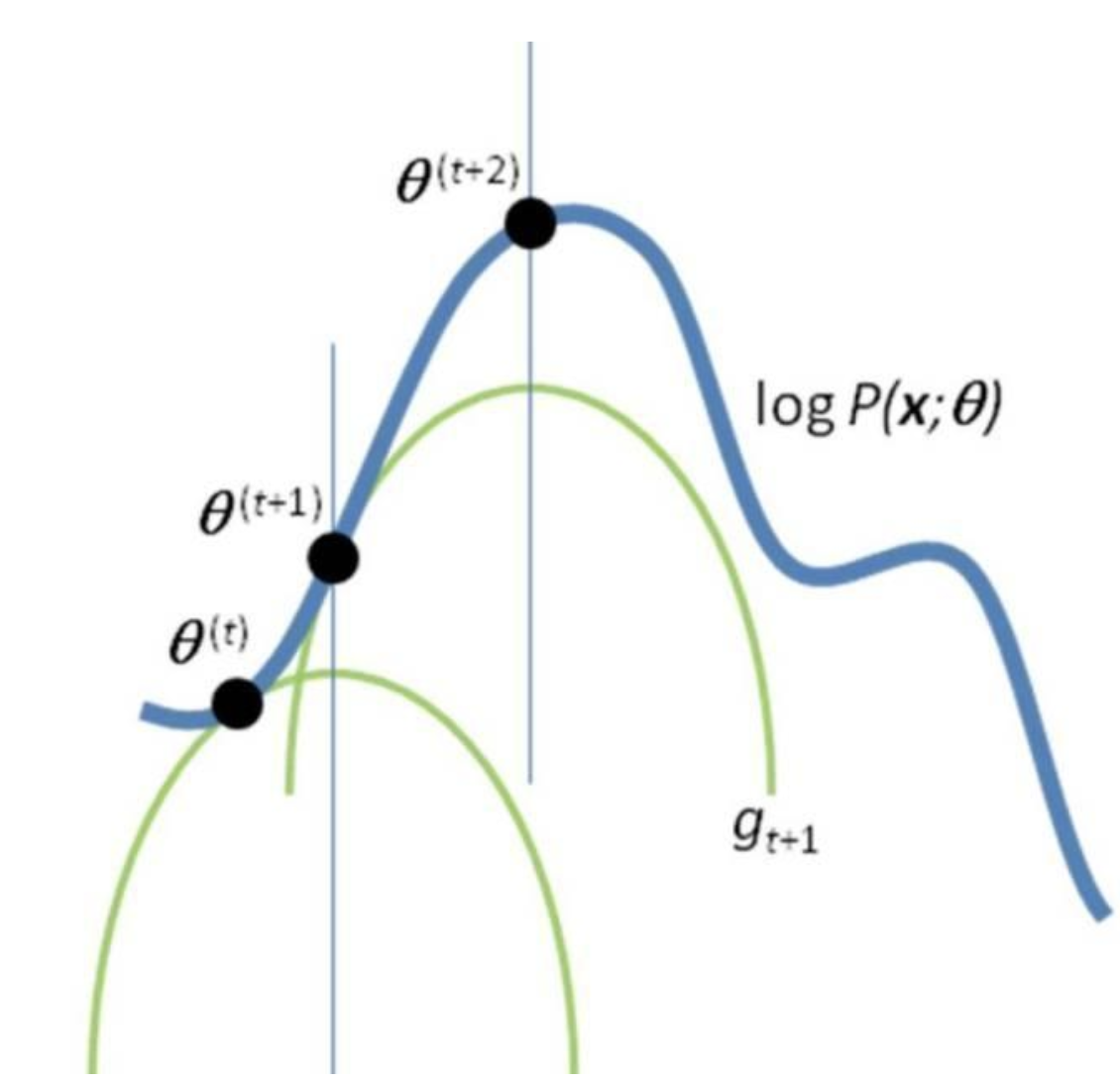EM算法可以视为通过不断构造局部下界函数来逼近最大似然函数的最大值。

$l\left(\theta \right)=logp\left(x|\theta \right)=log\int p\left(x,z|\theta \right)dz$${l(\theta) = log p(x|\theta) = log \int p(x,z|\theta) dz}$

$\begin{array}{rl}l\left(\theta \right)\ge & \int Q\left(z\right)log\frac{p\left(x,z|\theta \right)}{Q\left(z\right)}dz=\int Q\left(z\right)log\frac{p\left(z|x,\theta \right)p\left(x|\theta \right)}{Q\left(z\right)}dz\\ & =\int Q\left(z\right)logp\left(x|\theta \right)dz-\int Q\left(z\right)log\frac{p\left(z|x,\theta \right)}{Q\left(z\right)}dz\\ & =logp\left(x|\theta \right)+\int Q\left(z\right)log\frac{p\left(z|x,\theta \right)}{Q\left(z\right)}dz\phantom{\rule{1em}{0ex}}\left(\int Q\left(z\right)dz=1\right)\\ & =l\left(\theta \right)-KL\left(Q||p\left(z|x,\theta \right)\right)\end{array}$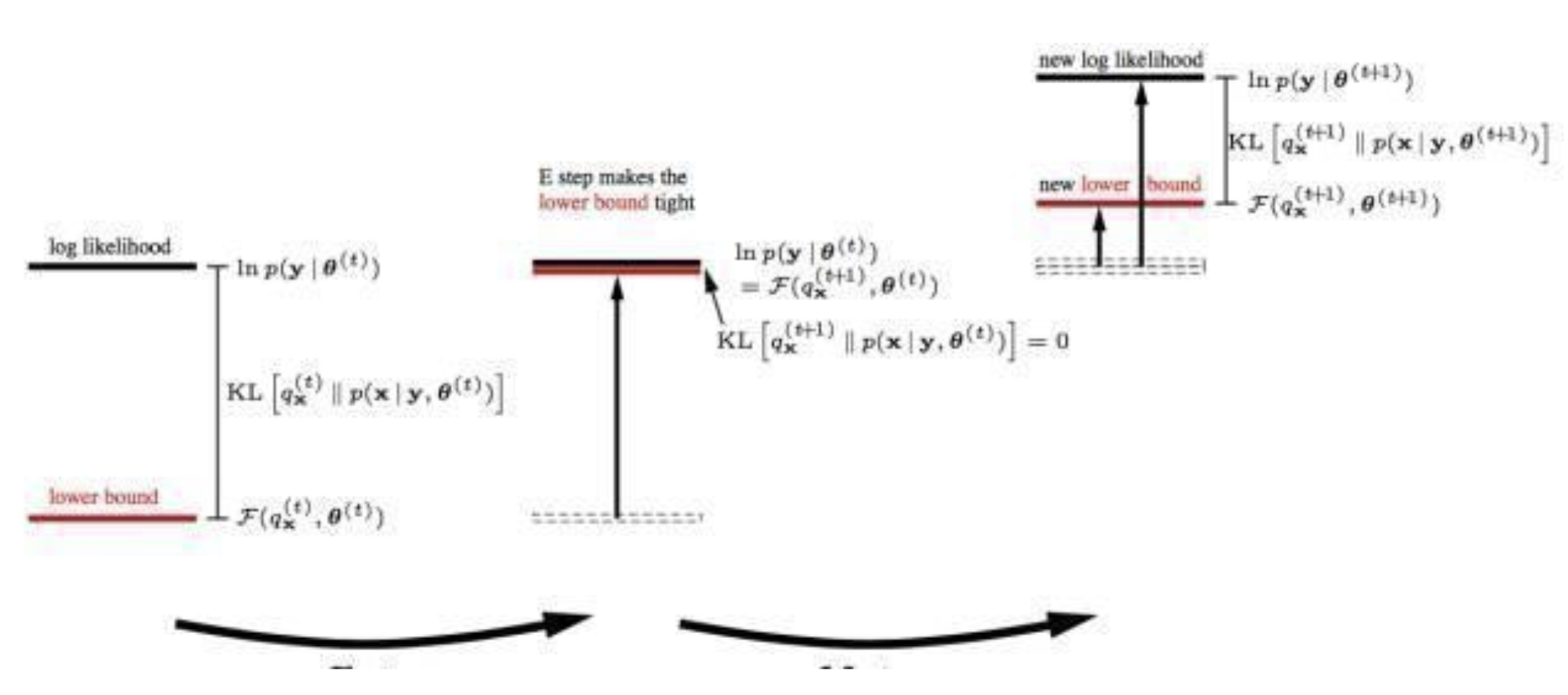2018-08-19 19:30:55 gq930901 阅读数 150
• ###### 人工智能之机器学习算法的介绍

4275 人正在学习 去看看 CSDN讲师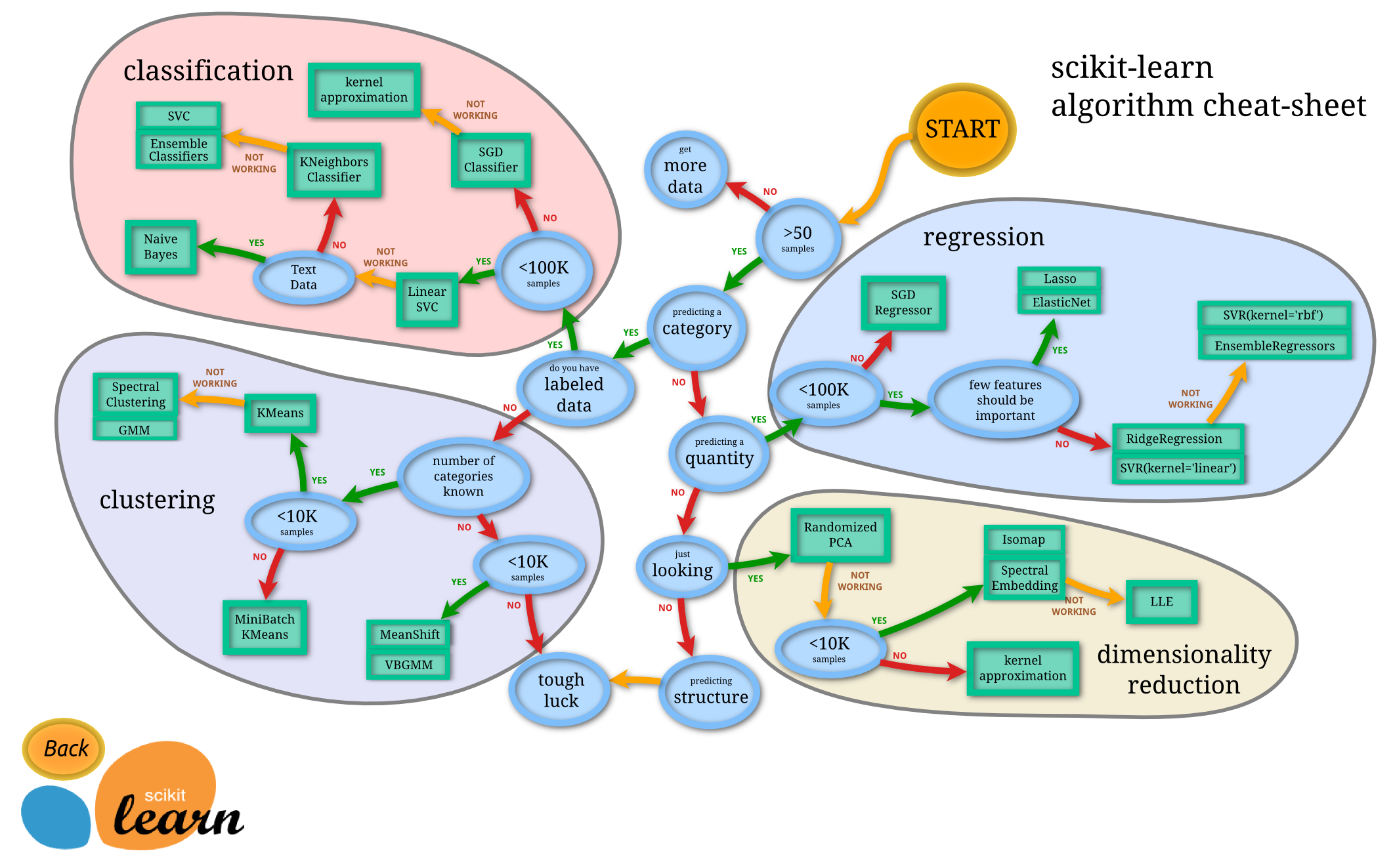1. 决策树
2. 随机森林算法
3. 逻辑回归
4. SVM
5. 朴素贝叶斯
6. K最近邻算法
7. K均值算法
9. 神经网络
10. 马尔可夫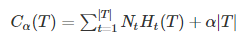决策树的剪枝过程（泛化过程）就是从叶子节点开始递归，记其父节点将所有子节点回缩后的子树为Tb（分类值取类别比例最大的特征值），未回缩的子树为Ta，如果Cα(Ta)≥Cα(Tb)说明回缩后使得损失函数减小了，那么应该使这棵子树回缩，递归直到无法回缩为止，这样使用“贪心”的思想进行剪枝可以降低损失函数值，也使决策树得到泛化。

CART（分类回归树）既可以用于分类也可以用于回归，回归问题中，CART使用平方误差最小化准则来选择特征并进行划分。每一个叶子节点给出的预测值，是划分到该叶子节点的所有样本目标值的均值，这样只是在给定划分的情况下最小化了平方误差。